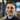Blog

# Declarative Programming with Prolog

February 11, 2018

Prolog is a declarative language around logic programming, where we can specify the rules and facts of a problem and let the language resolve the solution.

Sometimes requirements come up along the lines of “these are rules for discounts, this is a users’s info, purchase history, current inventory, what discounts are available to them?”, in an imperative world we write an algorithm describing how to answer that problem, but with prolog we can just describe the problem. This is somewhat similar to database query languages like SQL or Cypher, in that we describe what we want, but more general purpose in that we can declare logical functions.

Prolog facts in our example will come in two flavors, bound variables `A = 2`, and unbound variables `B`. The difference is that with the former there must be a variable A and it must equal 2, the latter only states that there is a variable B - letting Prolog assign value to it (this comes up in a second).

Prolog rules work in a few ways, one is defining an equation using the `:-` operator like `A :- B`. This type of equation is a little different in Prolog, in that both the left-hand side and the right-hand side must always balance. Prolog can assign values to the unbound variables to make the function balance. For example the function `A :- B + C.`, with a fact `A = 3, B = 2.`, Prolog will use the unbound variables to balance the equation, `C = 1` would be a valid solution to keep the equation to balance.

The other type of rule looks like `maplist(all_distinct, Rows)`, where we say given a list with the variable name Rows, all its elements must be distinct. Chaining these together starts to produce some really interesting solutions.

Under the hood Prolog goes through a depth first search of the problem space looking for candidate values that can balance the equation. For this article we will just be going through a Sodoku solver, because the rules are a little more straight forward and a solution is extremely easy to understand.

We’re going to use a package called CLP(FD) which makes it even easier, import it with `:- use_module(library(clpfd)).`

### Getting started

Our solver will take the form `sodoku(Rows) :- comma_seperated, list_of_rules, goes_here`, where the left-hand side is our 9x9 grid of facts with some bound and unbound variables, and the right hand side will be our rules. Let’s see what these rules look like:

#### Only contain numbers between 1-9

`append(Rows, Vs), Vs ins 1..9,` combine all the rows and ensure everything only includes integers between 1-9

#### Rows only have a single occurrence of a number

`maplist(all_distinct, Rows)` apply ‘all_distinct’ to the elements in the row (numbers can’t duplicate)

#### Columns only have a single occurrence of a number

`transpose(Rows, Columns), maplist(all_distinct, Columns)`, ‘transpose’ to get columns and do a similar ‘all_distinct’ check

#### 3x3 blocks only have a single occurrence of a number

This bit is a little tricky and uses recursion to solve for the blocks. We start by assigning each row to a variable name, so we pass them in groups of 3 to our recursive function.

``````Rows = [A,B,C,D,E,F,G,H,I],
blocks(A, B, C), blocks(D, E, F), blocks(G, H, I).``````

This function takes the first 3 variables of the list (giving us a 3x3 block), checks `all_distinct` on them, then passes the rest of the list to itself again.

``````blocks([A,B,C|Bs1], [D,E,F|Bs2], [G,H,I|Bs3]) :-
all_distinct([A,B,C,D,E,F,G,H,I]),
blocks(Bs1, Bs2, Bs3).``````

Since we use recursion we need an exit point when the lists are empty, we define `blocks([], [], []).`

### Putting it all together

``````:- use_module(library(clpfd)).

sudoku(Rows) :-
append(Rows, Vs), Vs ins 1..9,
maplist(all_distinct, Rows),
transpose(Rows, Columns),
maplist(all_distinct, Columns),
Rows = [A,B,C,D,E,F,G,H,I],
blocks(A, B, C), blocks(D, E, F), blocks(G, H, I).

blocks([], [], []).
blocks([A,B,C|Bs1], [D,E,F|Bs2], [G,H,I|Bs3]) :-
all_distinct([A,B,C,D,E,F,G,H,I]),
blocks(Bs1, Bs2, Bs3).``````

This is an example Sodoku for our input. We state a fact, `Rows = [...]` like, then pass that into out function and get a solution!

``````Rows = [
[_,_,7, _,_,3, _,5,_],
[_,3,_, 9,7,_, 4,_,8],
[8,9,_, 1,_,2, _,3,_],

[_,_,_, 7,_,_, 9,_,_],
[_,_,5, _,3,_, 8,_,6],
[_,_,8, _,_,_, 3,_,1],

[_,7,9, _,_,4, 1,_,_],
[4,8,1, _,9,_, _,_,2],
[6,_,_, _,8,_, _,_,_]
],
Rows = [A,B,C,D,E,F,G,H,I],
sudoku(Rows).``````Written by Andrew Klotz. Building tools to help people to do their best. You should follow him on Twitter